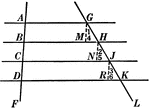### 4 Parallel Lines Cut By 2 Transversals

Illustration used to prove the theorem "If three or more parallel lines intercept equal segments on…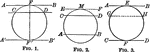### Circles With Parallels Intercepting Equal Arcs

Illustration of a circle with parallels intercepting equal arcs on a circumference.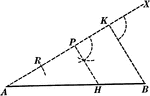### Line Divided Into Equal Parts

Illustration used to show how to divide a given straight line into any number of equal parts.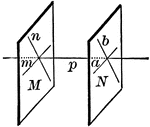### Planes Perpendicular To A Line

"Two planes perpendicular to the same straight line are parallel."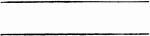### Parallel Lines

Illustration of parallel lines.### Parallel Lines

"Two straight lines perpendicular to the same plane are parallel."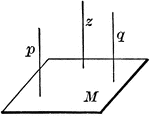### Parallel Lines

"Two straight lines that are parallel to a third are parallel to each other."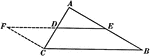### Line Joining Midpoints on a Triangle

Illustration used to prove "The line joining the mid-points of two sides of a triangle is parallel to…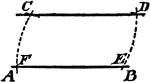### Construction Of A Parallel

An illustration showing the construction used to erect a parallel line. "With C as a center, draw the…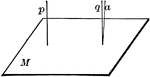### Lines Perpendicular To A Plane

"If one of two parallels is perpendicular to a plane, the other is also."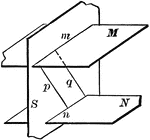### 3 Intersecting Planes

Illustration of three intersecting planes. "The intersections of two parallel planes by a third plane…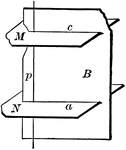### 3 Intersecting Planes

Illustration of three intersecting planes. "The straight line perpendicular to one of two parallel lanes…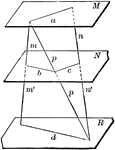### 3 Parallel Planes

"If two straight lines are cut by three parallel planes, the corresponding segments are proportional."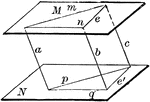### Angles In Parallel Planes

"If two angles not in the same plane have their sides respectively parallel and lying in the same direction,…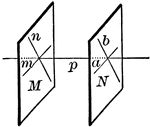### Parallel Planes

"If two intersecting lines are each parallel to a given plane, the plane of these lines is parallel…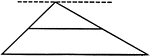### Line Bisecting Side of a Triangle

Illustration used to prove "The line bisecting one side of a triangle and parallel to another side bisects…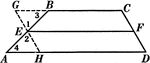### Line Bisecting Trapezoid Sides

Illustration used to prove the theorem "The line bisecting one of the non parallel sides of a trapezoid…